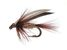cancel
Showing results for
Did you mean:New Contributor III

## Requesting help with units of angular dispersion for reflection grating

Dear ACS members,

Could someone please explain the units of angular dispersion for a reflection grating? The equation is Da = n / d * cos(r). An optics book listed the units: Da is angular dispersion (radians/nm), n is the reflection order (unitless), d is the spacing between rulings (nm), cos(r) is the cosine of the reflected angle, and r is the reflection angle (degrees). I do not understand where the radians come from in the result given the units of the calculation. This is the only book I could find the units specifically listed. Is this a misprint?

Thank you,

Jay Brown